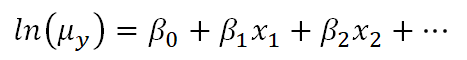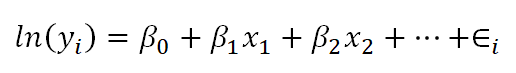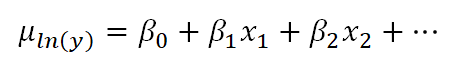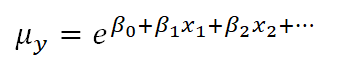### Count Models: Understanding the Log Link Function

November 12th, 2020 by

When we run a statistical model, we are in a sense creating a mathematical equation. The simplest regression model looks like this:

Yi = β0 + β1X+ εi

The left side of the equation is the sum of two parts on the right: the fixed component, β0 + β1X, and the random component, εi.

You’ll also sometimes see the equation written (more…)

### The Difference Between Link Functions and Data Transformations

September 24th, 2018 by

Generalized linear models—and generalized linear mixed models—are called generalized linear because they connect a model’s outcome to its predictors in a linear way. The function used to make this connection is called a link function. Link functions sounds like an exotic term, but they’re actually much simpler than they sound.

For example, Poisson regression (commonly used for outcomes that are counts) makes use of a natural log link function as follows:Clearly, there is not a direct linear relationship of the x variables to the average count, but there is a “sort of linear” relationship happening: a function of the mean of y is related to a linear combination of x variables. In other words, the linear model has now been generalized to a bigger type of situation.

This can lead to confusion, though, because on the surface it looks very similar to what happens when we transform the dependent variable in a linear model, like a linear regression.

The key thing to understand is that the natural log link function is a function of the mean of y, not the y values themselves.

### Transformations of Y

Below is a linear model equation where the original dependent variable, y, has been natural log transformed. That is, the natural log has been taken of each individual value of y, and that is being used as the dependent variable.The linear model with the log transformation is providing an equation for an individual value of ln(y). We could also write it as follows, where we are modeling the mean of ln(y) (note the error term is no longer present):This makes the difference a bit clearer. When we transform the data in a linear model, we are no longer claiming that y is normally distributed around a mean, given the x values — we are claiming that our new outcome variable, ln(yi), is normally distributed.

In fact, we often make this transformation specifically because the values of y do not appear to be normally distributed around their average.

In the case of the Poisson model, however, the link function does not change the distribution of the actual observations in some way to make them something other than Poisson distributed. Instead, the link function defines the relationship of the x variables directly to the mean of the Poisson distributed y. The individual observations then vary around this expected value accordingly.

### The mean of the log is not the log of the mean

As you may know, if you have used this kind of data transformation in a linear model before, you cannot simply take the exponent of the mean of ln(y) to get the mean of y.

You might be surprised to know, though, that you can do this with a link function. If you have specific values of your x variables, you can calculate the predicted average count, μy based on those x values by inversing the natural log:This ability to back-transform means (and regression coefficients) to a more intuitive scale is part of what makes generalized linear models so useful.

### Member Training: Count Models

March 3rd, 2015 by

Count variables are common dependent variables in many fields. For example:• Number of diseased trees
• Number of salamander eggs that hatch
• Number of crimes committed in a neighborhood

Although they are numerical and look like they should work in linear models, they often don’t.

Not only are they discrete instead of continuous (you can’t have 7.2 eggs hatching!), they can’t go below 0. And since 0 is often the most common value, they’re often highly skewed — so skewed, in fact, that transformations don’t work.

There are, however, generalized linear models that work well for count data. They take into account the specific issues inherent in count data. They should be accessible to anyone who is familiar with linear or logistic regression.

In this webinar, we’ll discuss the different model options for count data, including how to figure out which one works best. We’ll go into detail about how the models are set up, some key statistics, and how to interpret parameter estimates.

Note: This training is an exclusive benefit to members of the Statistically Speaking Membership Program and part of the Stat’s Amore Trainings Series. Each Stat’s Amore Training is approximately 90 minutes long.

Not a Member? Join!